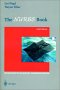# Maths - Curves and SurfacesGeometrys are usually created from polygons. The edges of these polygons are straight lines connecting up the vertex coordinates. How do we make curved, organic looking surfaces, normals help to reduce the look of the sharp edges but do not change the angular shape of the outline.

One way to do this is to generate curved surfaces mathematically by using the vertices as control points. There are a number of methods to do this as described in the following sections:

Another use for this type of curve fitting is in animation, where we want to change some variable such as position over time.

## Bezier Curves(cubic) Bezier curves can be generated recursively by joining up the midpoints of the lines joining the midpoints. The equation of this curve is given by:or

B(t) = (1-t)^3 p0 + 3t(1-t)^2 p1 + 3t^2(1-t) p2 + t^3 p3

## B-SplineThe generated curve does not necessarily pass through the control points, however if B-splines are calculated for adjacent sets of control points the curve segments will join up and produce a continuous surface. The equation for B-spline is:or

B(t) = 1/6 (-p0 + 3p1 -3p2+ p3)t^3 + 1/2 (p0 - 2p1 + p2) t^2 + 1/2 (-p0 + p2) t + 1/6 (p0 +4p1 + p2)

## NURBS (Non-Uniform Rational B-Spline)

This allows a curvy surface to be rendered directly, without first generating a tessellated surface. This uses control methods called evaluators. NURBS is supported by OpenGL.

### Modeling splines using Java

I don't know of any general purpose spline tools in Java, but there are spline tools for specific tasks:

If you want to draw a cubic curve in 2D you could use java.awt.geom.cubicCurve2D

If you want to move thorough 3D space using a splineInterpolator using Java3d then you could use the following utilities:

```com.sun.j3d.utils.behaviors.interpolators.RotPosScaleTCBSplinePathInterpolator
com.sun.j3d.utils.behaviors.interpolators.TCBKeyFrame
first you need a set of keyframes:
TCBKeyFrame[] splineKeyFrames = new TCBKeyFrame[nb];    for (int i = 0; i < nb; i++) {        splineKeyFrames[i] = new TCBKeyFrame((float)i/(nb - 1), 0, knots[i],r,                            s, 0.0f, 0.0f, 0.0f);    }The you can then put this into an interpolator and put this into the scenegraph:

TransformGroup target = new TransformGroup();
RotPosScaleTCBSplinePathInterpolator splineInterpolator =
new RotPosScaleTCBSplinePathInterpolator(myAlpha, target, new Transform3D(), splineKeyFrames);
```

 metadata block see also: Correspondence about this page Book Shop - Further reading. Where I can, I have put links to Amazon for books that are relevant to the subject, click on the appropriate country flag to get more details of the book or to buy it from them.Curves and Surfaces in Geometric Modeling: Theory and AlgorithmsThe NURBs Book (Monographs in Visual Communication) - A NURBS book for software developer, it covers the theory and also the practical side. Other Books about Curves and Surfaces

This site may have errors. Don't use for critical systems.